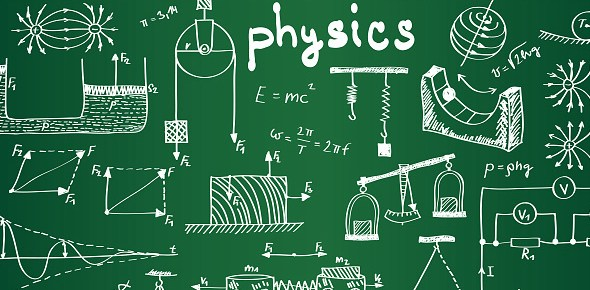# Physical Science Chapter 15

22 Questions | Attempts: 95
ShareSettingsPhysical science. Yes.

• 1.
The more you know about an object's position, the more you know about it's momentum.
• A.

True

• B.

False

• 2.
Matter has both wave an particle properties.
• A.

True

• B.

False

• 3.
Electrons can form interference patterns, just as light can.
• A.

True

• B.

False

• 4.
It is impossible to predict exactly where an electron will strike the screen after passing through two slits in a double-slit experiment.
• A.

True

• B.

False

• 5.
An object's momentum is the only property associated with probability waves.
• A.

True

• B.

False

• 6.
If moving slowly enough, small objects can have wavelengths that reach macroscopic dimensions.
• A.

True

• B.

False

• 7.
The likelihood of detecting a particle as it travels through space.
• 8.
The more you know about the position the less you know about momentum, and the more you know about momentum the less you know about position.
• 9.
Phenomenon that creates areas of high probability where the electron will strike the screen
• 10.
Phenomenon that creates areas of low probability where the electron is unlikely to strike the screen
• 11.
Postulates a universe where actions do not lead to pre-determined effects
• 12.
The physical world continues on a predetermined course once set in motion
• 13.
The likelihood of detecting a particle at a given location
• 14.
Louis de Brogile proposed that matter has the same _____________ duality as light
• 15.
To see interference patterns in an electron two-slit experiment, the slit's spacing needs to be about the same size as an electron's _____________
• 16.
The product of the uncertainty of an object's position and momentum is always greater than or equal to ______________
• 17.
When an electron is moving, it's ____________properties are manifest
• 18.
When an electron is detected, its _________properties are manifest
• 19.
If the speed of a particle increases, its wavelength
• A.

Decreases

• B.

Increases

• C.

Stays the same

• D.

Could increase, decrease, or stay the same

• 20.
Which of the following could not form a diffraction pattern in a double-slit experiment
• A.

Visible light

• B.

X-rays

• C.

Ultraviolet light

• D.

Electrons

• E.

All of the above could form diffraction patterns

• 21.
For which phenomenon would the Heisenberg Uncertainty Principle be a significant consideration in describing motion?
• A.

Electrons in atoms

• B.

Planets in orbit around the sun

• C.

The space shuttle in orbit around Earth

• D.

Billiard balls on a pool table

• E.

Brownian motion of dust particles in the air

• 22.
Macroscopic objects don't show interference effects because
• A.

Quantum mechanics doesn't apply to big objects

• B.

They are larger than the wavelength of light

• C.

Their wavelengths are too short

• D.

They can't fit through small slits

• E.

They don't have wave properties

## Related TopicsBack to top
×

Wait!
Here's an interesting quiz for you.